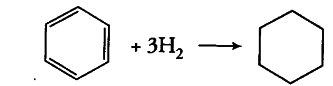# Use these data to estimate the magnitude of the resonance energy

The standard molar enthalpies of formation of cyclohexane (I) and benzene (l) at 25° C are - 156 and + 49 kJ /mol respectively. The standard enthalpy of hydrogenation of cyclohexene(Z) at 25°C is - 119 kJ /mol. Use these data to estimate the magnitude of the resonance energy of benzene.

Standard enthalpy of hydrogenation of cyclohexene = -119 kJ /mol. This is the observed enthalpy of hydrogenation of one double bond (present in cyclohexene). If benzene is considered as cyclohexatriene, the observed enthalpy of the reaction,